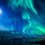An Observation by Myself !

This observation seems extremely intuitive, but is a lot helpful when dealing with most of the problems involving Second Principle of Mathematical Induction :-

$\boxed{a^{k}-b^{k} = (a^{k-n}-b^{k-n})(a^{n}+b^{n})-(ab)^{n}(a^{k-2n}-b^{k-2n})}$

I suggest the reader to accept the following problem as a challenge and post its solution in the comments ( It was this problem that made me realize about the identity) :-

INMO Type Problem :- Let $R = (5 \sqrt{5} + 11)^{2n+1}$ and let $f$ be the fractional part of $R$. Show that $Rf = 4^{2n+1}$.

By the way the above problem is not original.Note by Karthik Venkata
4 years, 6 months ago

This discussion board is a place to discuss our Daily Challenges and the math and science related to those challenges. Explanations are more than just a solution — they should explain the steps and thinking strategies that you used to obtain the solution. Comments should further the discussion of math and science.

When posting on Brilliant:

• Use the emojis to react to an explanation, whether you're congratulating a job well done , or just really confused .
• Ask specific questions about the challenge or the steps in somebody's explanation. Well-posed questions can add a lot to the discussion, but posting "I don't understand!" doesn't help anyone.
• Try to contribute something new to the discussion, whether it is an extension, generalization or other idea related to the challenge.
• Stay on topic — we're all here to learn more about math and science, not to hear about your favorite get-rich-quick scheme or current world events.

MarkdownAppears as
*italics* or _italics_ italics
**bold** or __bold__ bold

- bulleted
- list

• bulleted
• list

1. numbered
2. list

1. numbered
2. list
Note: you must add a full line of space before and after lists for them to show up correctly
paragraph 1

paragraph 2

paragraph 1

paragraph 2

> This is a quote
This is a quote
# I indented these lines
# 4 spaces, and now they show
# up as a code block.

print "hello world"
# I indented these lines
# 4 spaces, and now they show
# up as a code block.

print "hello world"
MathAppears as
Remember to wrap math in $$...$$ or $...$ to ensure proper formatting.
2 \times 3 $2 \times 3$
2^{34} $2^{34}$
a_{i-1} $a_{i-1}$
\frac{2}{3} $\frac{2}{3}$
\sqrt{2} $\sqrt{2}$
\sum_{i=1}^3 $\sum_{i=1}^3$
\sin \theta $\sin \theta$
\boxed{123} $\boxed{123}$

Sort by:

Great observation.

The $n = 1$ case is similar to what we get from Newton's Identities, namely

$a^k + b^k = ( a^{k-1} + b^{k-1} ) ( a + b ) - ( ab) ^ 2 ( a ^ {k-2} + b^ { k - 2 } ).$

Staff - 4 years, 6 months ago

Thanks Sir ! There is a really close connection between my observation and Newton's Identities, although they are used for completely different purposes !

- 4 years, 6 months ago

$R=I+f$

$0<5\sqrt 5 -11 <1$

Let $(5\sqrt 5 -11)^{2n+1}$=$f'$

Now , $I+f-f' = (5\sqrt 5+11)^{2n+1}-(5\sqrt 5 -11) ^{2n+1}= 2(^nC_1 (5\sqrt 5)^{2n}11 + ^n C3 (5\sqrt 5)^{2n-2}11^3 .............)$

Here RHS is integer So Lhs should be too so $f=f'$

Hence $Rf=Rf'=((5\sqrt 5 +11)^{2n+1}(5\sqrt 5 -11)^{2n+1}) = 4^{2n+1}$

- 4 years, 6 months ago

I think there is a fallacy :- $I + f - f' =$ an integer need not imply that $f = f'$. It may even be like $f = 1 + f'$ or $f = 2 + f'$ or generally $f = n + f'$ where $n$ is any integer, and still the fact that LHS ( as calculated by binomial expansion ) is an integer holds good.

- 4 years, 6 months ago

Hey man $f$ is fractional part of x hence $0 \le f<1$.Its a standard solution to these type of problems.

- 4 years, 6 months ago

I am not exposed to problems involving binomial expansion, sorry for troubling you ! I am getting confused....

- 4 years, 6 months ago

How do you say that RHS is an integer ? There is no proper reason for your claim. And also please do not include facts like Binomial Expansion, as the aim of this note is to deal with elegant proofs of theorems that are direct consequences of Induction Axioms.

- 4 years, 6 months ago

Can u tell me this question came in which yr in INMO.

- 4 years, 6 months ago

It says that this is an INMO-type problem, I guess it never actually came. However, I have a book (Problem-Solving Strategies by Arthur Engel), that has a problem which uses a very similar approach (adding the conjugate of the irrational number) for a certain problem.

In 1980, the oncoming IMO in Mongolia was cancelled on political grounds, Luxembourg hosted an Ersatz-IMO in Mersch. According to my book, that problem occured in this Ersatz-IMO in 1980. This was the problem:

Find the first digit before and after the decimal in: ${ (\sqrt { 2 } +\sqrt { 3 } ) }^{ 1980 }$

- 4 years, 6 months ago

No idea, there was no date in the book from which I came to know about the problem.

- 4 years, 6 months ago

RHS is an integer cause $5\sqrt 5$ always have even power and it is old IITJEE problem too.This method is absolutely correct .I subtracted those two terms to make RHS an integer.It is not a claim it is consequence of my subtraction

- 4 years, 6 months ago

Well thanks for explaining ! :)

- 4 years, 6 months ago